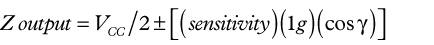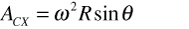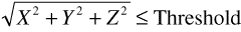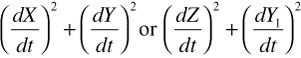# Using Dual-Axis Accelerometers to Protect Hard Disk Drives

### Introduction

Hard disk drives (HDDs) are becoming more widely used than ever before, due to the explosive growth in the introduction of portable equipment such as laptop computers, portable media players (PMPs), and handsets. As more and more devices incorporate HDDs, the need has become more pressing to protect them from shocks produced by severe impacts when a product that contains one is dropped accidentally. To increase the ability of HDDs to survive such events, their impact resistance must be enhanced.

There are two approaches to establishing the necessary impact resistance, active and passive.

Passive approaches have been in use for a long time; they simply cushion the device with impact-absorbing materials,1 usually rubber or gels. Gels, which tend to be better able to absorb an impact, are more widely used than rubber. However, gels cannot protect devices from damage caused by falls of more than one meter; this precludes their use in portable entertainment equipment. Devices such as handsets, MP3 players, and PMPs need to be protected for a drop of more than 1.5 meters (the average height of a human ear above the ground).

Among active approaches, there are two alternatives for protecting HDDs. One is to increase cache memory capacity so that the HDD is in a read or write mode less often. This approach would also reduce power consumption and heating. But it is costly and fails to deal with the impact that can occur should the HDD be in a read or write mode at the instant the fall begins. The second approach is to employ accelerometers (such as the Analog Devices ADXL320 dual-axis accelerometer, which measures axial acceleration) to detect a drop and then generate a signal that causes an HDD head to be recalled to a safe zone. If this can occur before the product hits the floor or other stationary surface, a collision between head and platter will be prevented. This approach was first used commercially in a notebook PC released by IBM in October 2003.

### Modeling Free Fall

The simplest model for free fall of an object is depicted in Figure 1, where the Z axis of the falling object is assumed to be perpendicular to the surface of the earth.Figure 1. Special case of the free-fall model—acceleration confined to a single axis.

In Figure 1(a) the object is assumed to be stationary, so that the accelerations along the X and Y axes are both zero, hence the force along the Z axis, governed by Newton’s second law, will have the value of 1 g (32.174 feet/second/second at sea level), corresponding to the stationary acceleration force due to gravity.

In Figure 1(b) the object is allowed to fall. The accelerations along the X and Y axes remain the same, zero g, but now the accelerometer that measures acceleration along the Z axis, being accelerated at the same rate as the object to which it is fastened, will record a value of zero g.

A more general case for a falling object is shown in Figure 2. Here the edges of the cube form arbitrary angles with regard to the orthogonal coordinate system.

In Figure 2(a), the object is depicted in a generalized arbitrary orientation; its edges form angles α, with respect to the X axis; β, with respect to the Y axis; and γ, with respect to the Z axis. At zero-g acceleration, the voltage output of each axis sensor is VCC/2. Accordingly, the outputs for the three axes will be:(1a)(1b)(1c)

“Sensitivity” refers to the output of the sensor per g. For the ADXL320, when powered by +3 V, the sensitivity will be 174 mV/g. If the direction of the detected linear acceleration corresponds with the positive direction of a coordinate axis—X, Y, or Z—its sign will be positive and its output will add to VCC/2; otherwise it will be negative and will subtract from VCC/2.

When the object is dropped suddenly, the accelerations along all three axes become zero because, regardless of the orientation of the object to the coordinate system, no acceleration will be detected along any axis since, as explained above, the accelerometer is accelerating towards the earth at the same rate as the falling body.

For portable equipment, we must also consider any angular acceleration that may be imparted to the object, as shown in Figure 3.

In order to simplify the calculation of the angular acceleration, the analysis will be confined to the plane determined by the X and Y axes, thereby simplifying the analysis.

If the angular velocity is ω and the radius of rotation is R, then the angular acceleration (AC) is:(2)

Therefore, the components of the angular acceleration along the X and Y axes will be:(3a)(3b)

So, in reality, the falling body will exhibit both linear acceleration and angular acceleration, a combination of the various cases discussed above.

To compute the time that will elapse when an object falls, starting with a velocity of zero perpendicular to the earth at the instant of the fall, we can use the following equation based upon Newton’s second law of motion:(4)

where h is the height of the fall and g is the gravitational acceleration, 32.174 feet/second/second.

To get a sense of the time available to respond to a fall, we can assume a height of 3 feet. Using equation (4), time = 432 ms.

Traditionally, the HDD protection algorithm has been based on free-fall modeling, as explained below, in which the outputs of the sensors contained in the accelerometers can be easily captured by a digital oscilloscope or other data sampling system.

A “test sled” can be assembled using two ADXL320 dual-axis accelerometers. The axes of the accelerometers are aligned with the X, Y, and Z axes, as depicted in Figure 4, thereby providing values of acceleration along the X, Y, and Z coordinates. (The Y1 output is redundant and is not used.) The outputs of the coordinate axes are sampled by a 12-bit ADC contained in an ADuC832 precision analog microcontroller, which integrates the sampled data and feeds it to an internal 8052-compatible core processor. The sampled data is then transferred to the computer—via an RS-232 interface—for analysis.

Figure 5 shows the sequence of responses sensed by the two sensors. Values X and Y are supplied by one accelerometer, the values Z and Y1 are supplied by the other accelerometer. Note also that the plot is divided into four consecutive intervals labeled: “static,” “rollover,” “free-fall drop,” and “impact.” The sampling interval, shown along the X axis, is determined by the ADC, which is clocked at 200 Hz for each variable, or one sample of each variable every 5 milliseconds. The Y-axis scale represents the values delivered by the 12-bit ADC in the ADuC832 smart-transducer front end, plotted for all four axes.

The test sled, placed at the edge of the table and caused to roll over, imparting angular acceleration—as depicted in Figure 4—produces the rollover data shown in Figure 5. (The Z-axis value, apparently not-equal-to-zero-g output in static mode, is caused by the unbalanced installation of the accelerometer.)

When the sled is pushed off the table, the values are all constant near their respective zero levels during this free-fall-drop interval, in line with the above assertion that during the free fall the outputs of all the accelerometers will be zero-g output.

(Note also that the zero-g output for the accelerometers along different axes in the same time interval is not quite the same.)

The traditional HDD protection algorithm is based on the data obtained in the arrangement just described. The system monitors the acceleration along the X, Y, and Z axes of the object. If the root-sum-of-squares value calculated from Equation 5 is equal to or less than the threshold value, a signal is sent to the computer associated with the HDD causing the head to park safely before the portable device collides with the floor.(5)

The choice of the threshold value is governed by the specific response-time requirement, as well as the sensor’s parameters—such as sensitivity, sensitivity change due to temperature, operation voltage, noise density, package alignment error, sensor resonant frequency and the working temperature range of the equipment. Normally, the threshold value can be determined from experiments, like the one described above. For example, a designer might choose a threshold value of 0.4 g.

### A New Differential Acceleration Algorithm

Now let’s look more closely at the behavior of the acceleration plots in Figure 5. If sufficient information to distinguish a fall were to be obtained during the rollover interval, there would be much more time available for protective action by the computer. In fact, the sensor outputs do vary during that interval, but the output values are not sufficiently pronounced to directly initiate the HDD protection process.

However, if a new function is formed, equal to the sum of the squares of the time derivatives of the X- and Y-axis accelerometer outputs (Equation 6),(6)

the results obtained will be as depicted in Figure 6. The values plotted in Figure 6 are the results of calculations based on outputs from the 12-bit ADC in the ADuC832 smart transducer front end. The sample numbers are again in 5-ms time increments. The black plot is the instant-by-instant value of (dX/dt)2 + (dY/dt)2, and the green plot is the instant-by-instant value of (dZ/dt)2 + (dY1/dt)2.Figure 6. Differential acceleration algorithm-time-derivative plots for (dX/dt)2 + (dY/dt)2 and (dZ/dt)2 + (dY1/dt)2.

As expected, the sums of the squares of the time derivatives are quite large during the rollover time interval, but they become quite small during the free-fall drop. This sequence of events can be employed to provide a reliable indication that a fall has occurred.

It is important to note that our research confirmed that either of the two accelerometers can be chosen, since they provide similar behavior. So the choice of the sensor axes to be monitored can be arbitrary.

We can now establish a new test algorithm, labeled “the differential acceleration algorithm” (Equation 7):(7)

The threshold of the time differential of sensor outputs, the key for the dropping detection, is related only to the sensitivity of the sensors. With the ADXL320, for example, the threshold can be selected as 200 counts (on the number scale used by the algorithm).

### Implementing the Differential Acceleration Algorithm

The principal components of a system to implement the differential acceleration algorithm are an ADXL320 dual-axis accelerometer, an AD8542 dual rail-to-rail amplifier and an ADuC832 smart transducer front end. A simplified schematic of the system appears in Figure 7.

The signals from the accelerometer are fed via the AD8542, which serves as a buffer between the accelerometer outputs and the ADuC832’s inputs, ADC0 and ADC1. A multiplexer switches between the two inputs at a rate of 200 samples per second for each channel, continuously monitoring the arriving acceleration signals.

The 8052 microcontroller core, which is a component of the ADuC832, implements the algorithm diagrammed in Figure 8. Then, whenever the system detects that a fall has occurred, a general I/O feeds an alert signal to the companion computer of the HDD so that the HDD will safely park the hard-disk-drive head before an impact occurs.

### Conclusion

One might ask whether a tri-axis sensor is essential for HDD protection. The answer is no. For, as demonstrated above, the use of an ADXL320 dual-axis accelerometer, when employed in a protection system that implements the differential acceleration algorithm discussed above,* performs the task quite ably. In addition to lower cost, the dual-axis sensor approach saves space and reduces power dissipation.

Based on the HDD protection system we built, it has been found that the response time between the instant a free fall occurs and the time the alert signal is generated will be 40 milliseconds, with a sampling rate of 200 samples per second per channel and a sensor bandwidth of 100 Hz. The time required to park a hard-disk-drive head should not exceed 150 ms in order to lower the cost of the whole system. Therefore, the total time from a detected free fall to the completion of the parking is no more than 190 ms. This is far less than the 432 ms that it takes for a portable product to fall 3 feet.

The algorithm described in this article is applicable for almost all the cases described above. The only case that it cannot detect is a free-fall event in which the time derivatives of the squares of the sensed X and Y accelerations at the instant that the fall occurs remain negligible during the free-fall drop. But this is quite unlikely, and, in our experience, it has never occurred.

The Analog Devices ADXL320 is a low-cost, low-power, dual-axis acceleration measuring system with signal-conditioned voltage outputs, all on a single monolithic IC. The product measures acceleration with a full-scale range of ±5 g (typical). The ADXL320 is housed in a very-thin, 4-mm × 4-mm × 1.45-mm, 16-lead plastic LFCSP.

The accelerometer contains a sensor and signal-conditioning circuitry to implement an open-loop acceleration measurement architecture. The output signals are two analog voltages proportional to the orthogonal accelerations.

The sensor is a polysilicon, surface-micromachined structure built on top of a silicon wafer. Polysilicon springs suspend the structure over the surface of the wafer and provide resistance against acceleration forces. Deflection of the structure is measured using a differential capacitor formed by fixed independent plates in relation to plates attached to the moving mass.

The fixed plates are driven by square waves which are 180° out of phase. When the device is subjected to an acceleration force, the beams deflect, unbalancing the differential capacitor—which results in an output square wave with amplitude proportional to acceleration. Phase-sensitive demodulation circuitry, contained in the demodulator block in the illustration, is then used to rectify the signal and determine whether the acceleration is positive or negative.

The demodulator’s measured accelerations, along the X axis and Y axis of the ADXL320, are amplified by the output amplifiers and brought off-chip through 32-kΩ resistors, as shown in the illustration. External capacitors can be used to provide filtering.

ADXL Low-g Accelerometer Selection Table part 1

ADXL Low-g Accelerometer Selection Table part 2

*Sensor bandwidth is set by the customer in the application.

### References

1Naoki Asakawa. “Tech Analysis: HDD for Mobile Phones Withstand 1.5-Meter Drop.” Nikkei Electronics Asia, Jan 2005.

*Patent applied for.

### Acknowledgements

The authors would like to thank Harvey Weinberg and Christophe Lemaire for their technical expertise. The authors also would like to thank Justin Littlefield and Steve Grossman for assistance in writing this article.